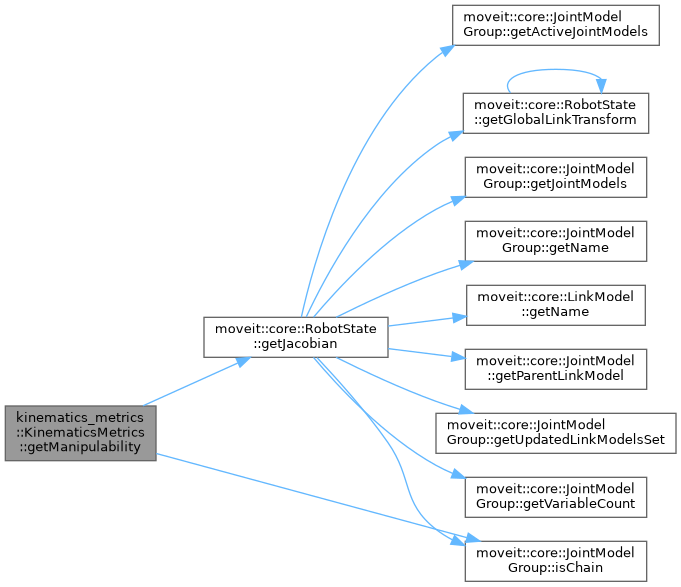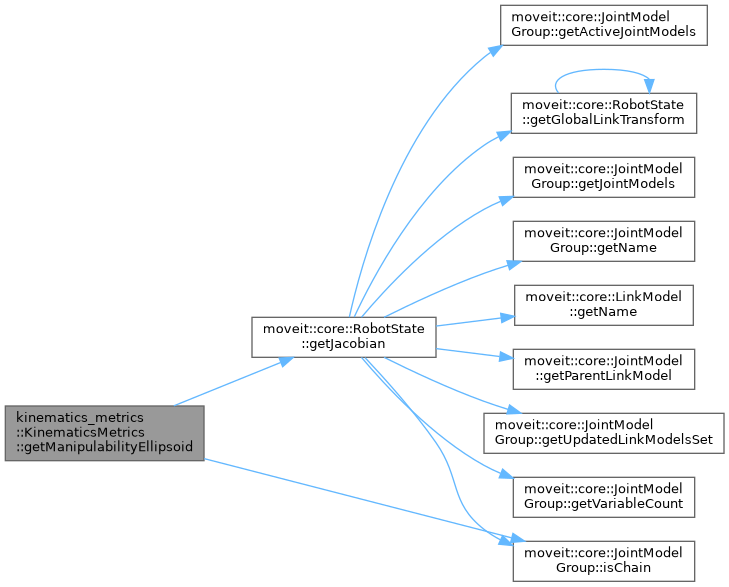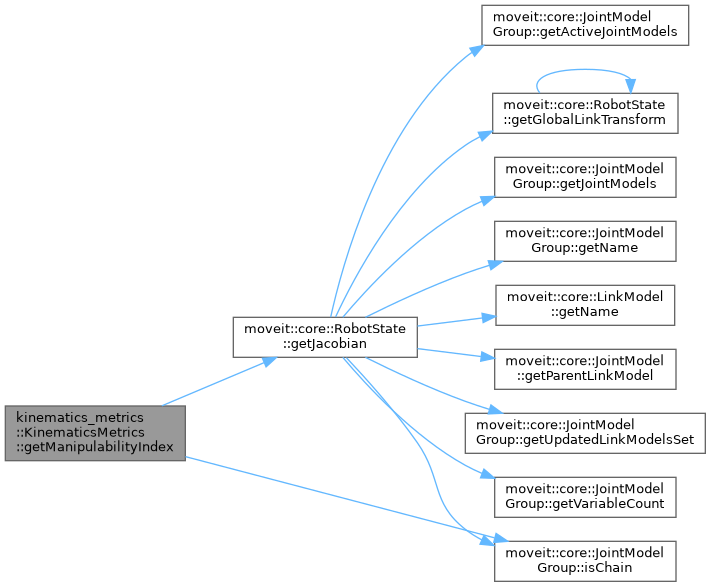moveit2 The MoveIt Motion Planning Framework for ROS 2.
kinematics_metrics::KinematicsMetrics Class Reference

Compute different kinds of metrics for kinematics evaluation. Currently includes manipulability. More...

`#include <kinematics_metrics.h>`

## Public Member Functions

KinematicsMetrics (const moveit::core::RobotModelConstPtr &robot_model)
Construct a KinematicsMetricss from a RobotModel. More...

bool getManipulabilityIndex (const moveit::core::RobotState &state, const std::string &group_name, double &manipulability_index, bool translation=false) const
Get the manipulability for a given group at a given joint configuration. More...

bool getManipulabilityIndex (const moveit::core::RobotState &state, const moveit::core::JointModelGroup *joint_model_group, double &manipulability_index, bool translation=false) const
Get the manipulability for a given group at a given joint configuration. More...

bool getManipulabilityEllipsoid (const moveit::core::RobotState &state, const std::string &group_name, Eigen::MatrixXcd &eigen_values, Eigen::MatrixXcd &eigen_vectors) const
Get the (translation) manipulability ellipsoid for a given group at a given joint configuration. More...

bool getManipulabilityEllipsoid (const moveit::core::RobotState &state, const moveit::core::JointModelGroup *joint_model_group, Eigen::MatrixXcd &eigen_values, Eigen::MatrixXcd &eigen_vectors) const
Get the (translation) manipulability ellipsoid for a given group at a given joint configuration. More...

bool getManipulability (const moveit::core::RobotState &state, const std::string &group_name, double &condition_number, bool translation=false) const
Get the manipulability = sigma_min/sigma_max where sigma_min and sigma_max are the smallest and largest singular values of the Jacobian matrix J. More...

bool getManipulability (const moveit::core::RobotState &state, const moveit::core::JointModelGroup *joint_model_group, double &condition_number, bool translation=false) const
Get the manipulability = sigma_min/sigma_max where sigma_min and sigma_max are the smallest and largest singular values of the Jacobian matrix J. More...

void setPenaltyMultiplier (double multiplier)

double getPenaltyMultiplier () const

## Protected Attributes

moveit::core::RobotModelConstPtr robot_model_

## Detailed Description

Compute different kinds of metrics for kinematics evaluation. Currently includes manipulability.

Definition at line 50 of file kinematics_metrics.h.

## ◆ KinematicsMetrics()

 kinematics_metrics::KinematicsMetrics::KinematicsMetrics ( const moveit::core::RobotModelConstPtr & robot_model )
inline

Construct a KinematicsMetricss from a RobotModel.

Definition at line 54 of file kinematics_metrics.h.

## ◆ getManipulability() [1/2]

 bool kinematics_metrics::KinematicsMetrics::getManipulability ( const moveit::core::RobotState & state, const moveit::core::JointModelGroup * joint_model_group, double & condition_number, bool translation = `false` ) const

Get the manipulability = sigma_min/sigma_max where sigma_min and sigma_max are the smallest and largest singular values of the Jacobian matrix J.

Parameters
 state Complete kinematic state for the robot joint_model_group A pointer to the desired joint model group condition_number Condition number for JJ^T
Returns

Definition at line 221 of file kinematics_metrics.cpp.

Here is the call graph for this function:## ◆ getManipulability() [2/2]

 bool kinematics_metrics::KinematicsMetrics::getManipulability ( const moveit::core::RobotState & state, const std::string & group_name, double & condition_number, bool translation = `false` ) const

Get the manipulability = sigma_min/sigma_max where sigma_min and sigma_max are the smallest and largest singular values of the Jacobian matrix J.

Parameters
 state Complete kinematic state for the robot group_name The group name (e.g. "arm") condition_number Condition number for JJ^T
Returns

Definition at line 207 of file kinematics_metrics.cpp.

## ◆ getManipulabilityEllipsoid() [1/2]

 bool kinematics_metrics::KinematicsMetrics::getManipulabilityEllipsoid ( const moveit::core::RobotState & state, const moveit::core::JointModelGroup * joint_model_group, Eigen::MatrixXcd & eigen_values, Eigen::MatrixXcd & eigen_vectors ) const

Get the (translation) manipulability ellipsoid for a given group at a given joint configuration.

Parameters
 state Complete kinematic state for the robot joint_model_group A pointer to the desired joint model group eigen_values The eigen values for the translation part of JJ^T eigen_vectors The eigen vectors for the translation part of JJ^T
Returns

Definition at line 188 of file kinematics_metrics.cpp.

Here is the call graph for this function:## ◆ getManipulabilityEllipsoid() [2/2]

 bool kinematics_metrics::KinematicsMetrics::getManipulabilityEllipsoid ( const moveit::core::RobotState & state, const std::string & group_name, Eigen::MatrixXcd & eigen_values, Eigen::MatrixXcd & eigen_vectors ) const

Get the (translation) manipulability ellipsoid for a given group at a given joint configuration.

Parameters
 state Complete kinematic state for the robot group_name The group name (e.g. "arm") eigen_values The eigen values for the translation part of JJ^T eigen_vectors The eigen vectors for the translation part of JJ^T
Returns

Definition at line 173 of file kinematics_metrics.cpp.

## ◆ getManipulabilityIndex() [1/2]

 bool kinematics_metrics::KinematicsMetrics::getManipulabilityIndex ( const moveit::core::RobotState & state, const moveit::core::JointModelGroup * joint_model_group, double & manipulability_index, bool translation = `false` ) const

Get the manipulability for a given group at a given joint configuration.

Parameters
 state Complete kinematic state for the robot joint_model_group A pointer to the desired joint model group manipulability_index The computed manipulability = sqrt(det(JJ^T))
Returns

Definition at line 112 of file kinematics_metrics.cpp.

Here is the call graph for this function:## ◆ getManipulabilityIndex() [2/2]

 bool kinematics_metrics::KinematicsMetrics::getManipulabilityIndex ( const moveit::core::RobotState & state, const std::string & group_name, double & manipulability_index, bool translation = `false` ) const

Get the manipulability for a given group at a given joint configuration.

Parameters
 state Complete kinematic state for the robot group_name The group name (e.g. "arm") manipulability_index The computed manipulability = sqrt(det(JJ^T))
Returns

Definition at line 98 of file kinematics_metrics.cpp.

## ◆ getPenaltyMultiplier()

 double kinematics_metrics::KinematicsMetrics::getPenaltyMultiplier ( ) const
inline

Definition at line 132 of file kinematics_metrics.h.

## ◆ setPenaltyMultiplier()

 void kinematics_metrics::KinematicsMetrics::setPenaltyMultiplier ( double multiplier )
inline

Definition at line 127 of file kinematics_metrics.h.

## ◆ robot_model_

 moveit::core::RobotModelConstPtr kinematics_metrics::KinematicsMetrics::robot_model_
protected

Definition at line 138 of file kinematics_metrics.h.

The documentation for this class was generated from the following files: